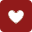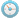×

×
• 剂型：
更多收起• 处方|医保：
• 厂家首字母：
• A
• B
• C
• D
• E
• F
• G
• H
• I
• J
• K
• L
• M
• N
• O
• P
• Q
• R
• S
• T
• U
• V
• W
• X
• Y
• Z
•磷酸哌嗪宝塔糖[甲]/0.2g*20粒
四川德元药业集团
规格： 0.2g*20粒
效期： 件装量：400到货通知
-
+
•●磷酸哌嗪宝塔糖[甲]/0.2g*30粒
江苏黄河
规格： 0.2g*30粒
效期： 件装量：200到货通知
-
+
月销:3+